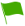# C3.2 The Mole

1.What is the name given to the amount of substance contained in one liter of a solution?

2.What is the name given to the volume that anyone mole of any gas occupies at S.T.P [standard temperature and pressure] and this volume is 22.4 liters?

3.Why is the hydrogen atom not a preferable atom of choice to be used as a standard atom to compare it to other atomic masses of other elements?

4.A solution whose concentration (molarity) is known is known as

5.A solution that contains one mole of a substance dissolved in one liter (dm3) of a solution is known as

6.The Avogadro’s constant (NA) = 6.02 × 1023 True or False

7.To find the volume of unknown concentration using volumes of standard solutions that react together is known as

8.Which of the following statement about Mono-atomic gases is correct

9.Define the relative atomic mass (R.A.M) of an element

10.State Gay-Lussac’s Law

11.The molarity of a substance shows how much the substance in terms of moles has been dissolved in one liter (dm3) of a solution

12.Mention the 2 ways in which chemical reactions can be expressed

13.In an ionic equation, what is the name given to the ions which never take part in the reaction

14.In an ionic equation, what is the name given to the ions which are converted to products and they hence take part in the reaction

15.The formula which expresses the actual number of each kind of atoms of each element present in the molecule is known as

16.Mention the number of atoms found in each R.A.M OR R.M.M of an element

17.An equation whereby the mole ratio of the reactants and the products is represented by whole numbers is called

18.The number of atoms of the element that join up to form a molecule of the element is known. as the atomicity of the given gas i.e. atomicity of chlorine gas is 2. True or Talse

19.Is the following equation TRUE OR FALSE? R.A.M =

20.Mention the 2 ways in which the concentration of a solution can be expressed

21.The simplest formula of a compound which expresses its percentage composition by mass is known as

22.A mole is the number of particles in the relative atomic mass of an element or the relative molecular mass of a substance expressed in grams. True or False

23.Empirical formula × N = MOLECULAR FORMULA; where N= simple whole number. True or False

24.Is the following equation CORRECT OR NOT?

25.Which of the following statement is correct about diluting a solution

26.Mentioned the 3 conditions that make an ionic equation written by a student absolutely correct

27.Mention the 2 common types of titration